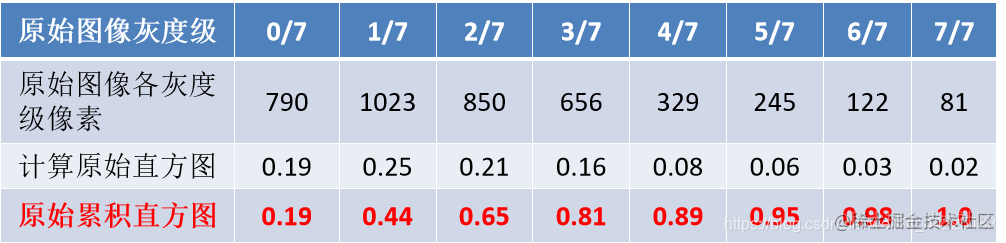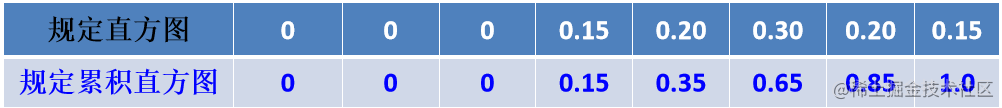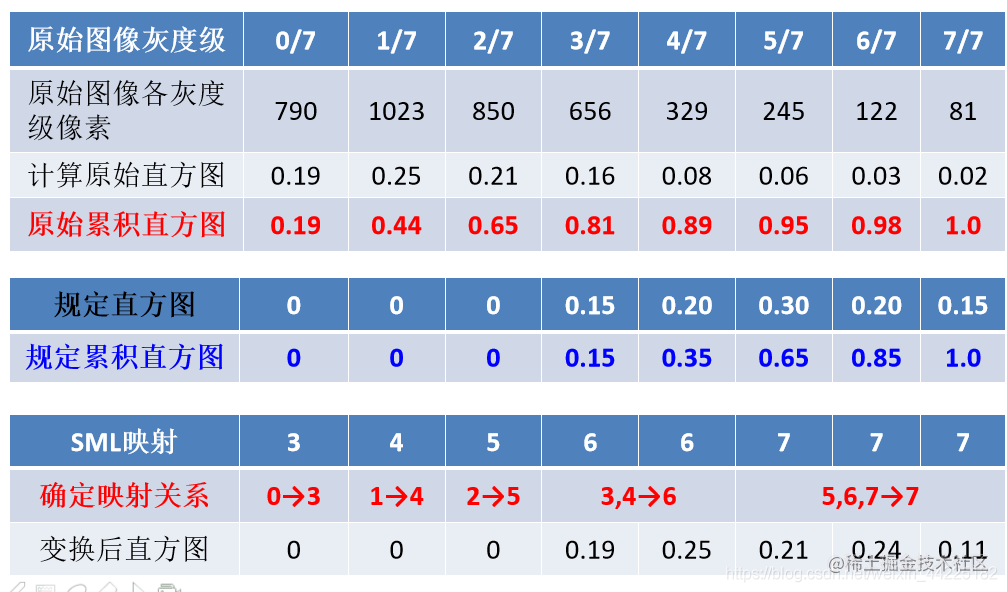# MATLAB--数字图像处理 图像直方图规定化

「这是我参与11月更文挑战的第14天，活动详情查看：2021最后一次更文挑战

# 前言

Hello！小伙伴！

## 直方图规定化

1. 分别计算出原图像和规定图像的累加直方图
2. 利用SML或者GML映射灰度值
3. 利用更新后的映射表转换原图像的灰度值

1. 这里是直接给出的原图像各灰度的像素个数，为了得到我们需要的直方累加图（其实是概率累加值，当然也可以不用概率，直接用像素点数也行，就是看起来数字比较大），需要先算出原图像各灰度的概率（占总像素个数的比例），然后在计算累加值2. 根据给出的规定直方图，同样的方法计算累加直方图，见下图3. 利用SML映射规则得出结果4. 利用映射变化表，更新原图像

SML规则懂了，GML规则也就好理解了(GML规则其实编程更难)：

SML中，我们是依次遍历原图累加直方图，在规定累加直方图寻找最靠近自己的。在GML(组映射)中，就变成了依次从规定累加直方图中，对比原图累加直方图，也是找到最靠近的值，进行灰度变换，只是这里的变换规则变了。具体举例来说，从上图看，这里我们这里从规定累加直方图的0.15看，前面的0其实不用看，再从原始累加直方图找到最接近0.15的值，是0.19，那么0.19对应和它对应灰度值前面的灰度变成规定累加直方图中0.15所对应的灰度值：3；第二个看规定累加直方图的0.35，靠近原图的0.44，那么原图的1变成4（假设这里0.44对应的原图灰度值为4，那么在前一个不为0和4之间变成4） 这里有的不好理解，举个例子： 原数组：1 0 0 2 0 3 0 0 0 4 0 0 6 GML映射变化规则的目标数组就是：1 2 2 2 3 3 4 4 4 4 6 6 6 解释：1前面的数变为1,1和2之间变成2,2和3之间变成3，3和4之间变成4,4和6之间变成6,简单的说就是变0变成后面最靠近的一个不为0的数。（这里算法自己需要掌握，即如何从原数组变成目标数组，这里先给个C++的验证算法）

``````#include<iostream>
using namespace std;
int main()
{
int t={2,0,1,0,3,0,0,6};
int i,j;
int tem;
int flag=0;
for (i=0;i<8;i++)
{
if(t[i]==0)
{

for(j=i;j<8;j++)
{
if(t[j]!=0)
{
tem=t[j];
break;}
}
t[i]=tem;

}
}
for(i=0;i<8;i++)
cout<<t[i]<<" ";
return 0;
}

MATLAB实战 原理懂了，肯定就是要开始实战了啊！ 这里我先上全部代码：

`````` t=imread('a1.jpg')
%获取图片的长和宽，用于计算总像素，即m*n
[m,n]=size(t);

%n_1 这里是先统计各灰度的像素数，后面会变换为概率
n_1=zeros(1,256);

%统计各灰度的像素数
for i=1:m
for j=1:n
n_1(t(i,j)+1)=n_1(t(i,j)+1)+1;
end
end

%转换为概率
n_1=n_1/m/n; %计算各个灰度级的概率

%p_1  记录原图的累积直方图概率
p_1=zeros(1,256);

%p_1 计算原始累积直方图的概率
for i=1:256
for j=1:i
p_1(i)=p_1(i)+n_1(j);
end
end

%n_2  规定直方图的灰度概率分布
n_2=[zeros(1,50),0.1,zeros(1,50),0.2,zeros(1,50),0.3,zeros(1,50),0.2,zeros(1,20),0.1,zeros(1,30),0.1];

%p_2 记录规定直方图的累积概率
p_2=zeros(1,256);

% 计算规定直方图累积概率
for i=1:256
for j=1:i
p_2(i)=p_2(i)+n_2(j);
end
end

%SML映射算法
data_1=zeros(1,256);%data_1 SML映射表

for i=1:256
min=abs(p_1(i)-p_2(1));
for j=2:256
if  abs(p_1(i)-p_2(j))<min
min=abs(p_1(i)-p_2(j));
data_1(i)=j-1;
end
end
end

%GML
data_2=zeros(1,256); %data_2记录GML映射表

for i=1:256
if n_2(i)~=0
tem=1;
min=abs(p_2(i)-p_1(1));
for j=2:256
if abs(p_2(i)-p_1(j))<min
min=abs(p_2(i)-p_1(j));
tem=j;
end
end
data_2(tem)=i-1;
end
end

%将上面得到的data_2 转换为目标数组（原理解释有c++算法）
for i=1:256
if data_2(i)==0
for j=i:256
if data_2(j)~=0
tem=data_2(j);
break;
end
end
data_2(i)=tem;
end
end

%利用SML、GML映射表，转换原图像
t2=t;
t3=t;

%转换算法
for i=1:m
for j=1:n
t2(i,j)=data_1(t(i,j)+1);
end
end
for i=1:m
for j=1:n
t3(i,j)=data_2(t(i,j)+1);
end
end

%显示
subplot(3,2,1),imshow(t),title('原图')
subplot(3,2,2),imhist(t),title('原图')
subplot(3,2,3),imshow(t2),title('SML')
subplot(3,2,4),imhist(t2),title('SML')
subplot(3,2,5),imshow(t3),title('GML')
subplot(3,2,6),imhist(t3),title('GML')

SML、GML两个算法用来一天才搞清楚原理，书上的公式开始看真的是好难啊，看不进去，网上的方法，真的是，没有自己想要的。算法其实一天就搞定了，当时由于MATLAB语法还不是很熟，走了很多弯路。下面总结下自己的踩坑吧：

• MATLAB里面的循环写法 for i=1:256 c++:for(i=1;i<=256;i++)

• for、if等用end结束，end个数必须和if、for配对，比如，3个for，2个if，就必须有5个end结束，不然程序会一直执行下去

• 程序语句最后有无 ； 的区别：写了 ； 本地代码区不会显示具体数据，反之不写，则会显示，建议还是写吧，和c++语法类似。

• 多打印数据，一步一步调试，便于寻找bug